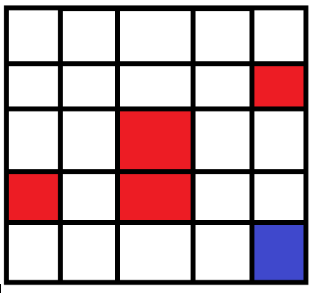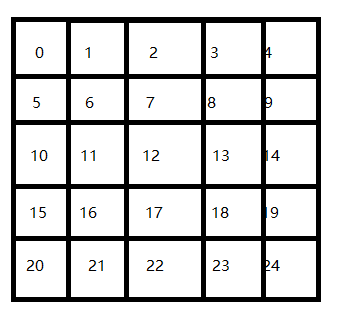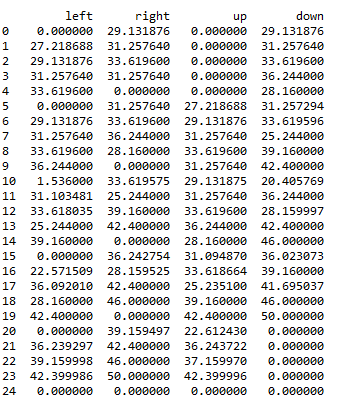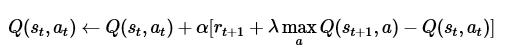## 一些说明、参阅

https://github.com/MorvanZhou/Reinforcement-learning-with-tensorflow/blob/master/contents/1_command_line_reinforcement_learning/treasure_on_right.py

https://github.com/simoninithomas/Deep_reinforcement_learning_Course/blob/master/Q%20learning/FrozenLake/Q%20Learning%20with%20FrozenLake.ipynb

https://www.cnblogs.com/hhh5460/p/10134018.html

http://baijiahao.baidu.com/s?id=1597978859962737001&wfr=spider&for=pc

https://www.jianshu.com/p/29db50000e3f

## 问题提出在机器人实际选择路径时，按照该表中的最大值选择，最终走到24号位置，并避开了红色方块。

## Q-learning的想法

### 具体公式在每次行动后，根据奖惩情况，更新该表，完成学习过程。在实现过程中，将奖惩情况也编制成一张表。表格式如上图类似。（该式子在很多网页文本中并没有固定的格式，如贪婪系数，在有些时候是随着步数的增加而递减的（可能）。

推荐阅读：

https://www.jianshu.com/p/29db50000e3f

等，其中包括了更新Q表中的一些过程。

## 代码实现-准备过程

```import numpy as np
import pandas as pd
import time
```

```N_STATES = 25   # the length of the 2 dimensional world
ACTIONS = ['left', 'right','up','down']     # available actions
EPSILON = 0.3   # greedy police
ALPHA = 0.8     # learning rate
GAMMA = 0.9    # discount factor
MAX_EPISODES = 100   # maximum episodes
FRESH_TIME = 0.00001    # fresh time for one move
```

### 创建Q表的函数：

```def build_q_table(n_states, actions):
table = pd.DataFrame(
np.zeros((n_states, len(actions))),     # q_table initial values
columns=actions,    # actions's name
)
return table
```

### 行为选择的函数：

```def choose_action(state, q_table):
state_actions = q_table.iloc[state, :]
if (np.random.uniform() > EPSILON) or ((state_actions == 0).all()):  # act non-greedy or state-action have no value
if state==0:
action_name=np.random.choice(['right','down'])
elif state>0 and state<4:
action_name=np.random.choice(['right','down','left'])
elif state==4:
action_name=np.random.choice(['left','down'])
elif state==5 or state==15 or state==10 :
action_name=np.random.choice(['right','up','down'])
elif state==9 or state==14 or state==19 :
action_name=np.random.choice(['left','up','down'])
elif state==20:
action_name=np.random.choice(['right','up'])
elif state>20 and state<24:
action_name=np.random.choice(['right','up','left'])
elif state==24:
action_name=np.random.choice(['left','up'])
else:
action_name=np.random.choice(ACTIONS)
else:   # act greedy
action_name = state_actions.idxmax()    # replace argmax to idxmax as argmax means a different function in newer version of pandas
return action_name
```

### 奖赏表达：

```def get_init_feedback_table(S,a):
tab=np.ones((25,4))
tab=-10;tab=-10;tab=-10
tab=-10;tab=-10;tab=-10;tab=-10
tab=-10;tab=-10;tab=-10;
tab=-10;tab=-10;tab=-1;tab=-10
tab=50;tab=50
return tab[S,a]
```

### 获取奖惩：

```def get_env_feedback(S, A):
action={'left':0,'right':1,'up':2,'down':3};
R=get_init_feedback_table(S,action[A])
if (S==19 and action[A]==3) or (S==23 and action[A]==1):
S = 'terminal'
return S,R
if action[A]==0:
S-=1
elif action[A]==1:
S+=1
elif action[A]==2:
S-=5
else:
S+=5
return S, R
```

## 代码实现-开始训练

```q_target = R + GAMMA * q_table.iloc[S_, :].max()
q_table.loc[S, A] += ALPHA * (q_target - q_table.loc[S, A])
```

这两行完成q表的更新。（对照贝尔曼方程）

```def rl():
# main part of RL loop
q_table = build_q_table(N_STATES, ACTIONS)
for episode in range(MAX_EPISODES):
S = 0
is_terminated = False

while not is_terminated:
A = choose_action(S, q_table)
S_, R = get_env_feedback(S, A)  # take action & get next state and reward
if S_ != 'terminal':
q_target = R + GAMMA * q_table.iloc[S_, :].max()   # next state is not terminal
else:
print(1)
q_target = R     # next state is terminal
is_terminated = True    # terminate this episode

q_table.loc[S, A] += ALPHA * (q_target - q_table.loc[S, A])  # update
S = S_  # move to next state
return q_table

if __name__ == "__main__":
q_table = rl()
print('\r\nQ-table:\n')
print(q_table)
```